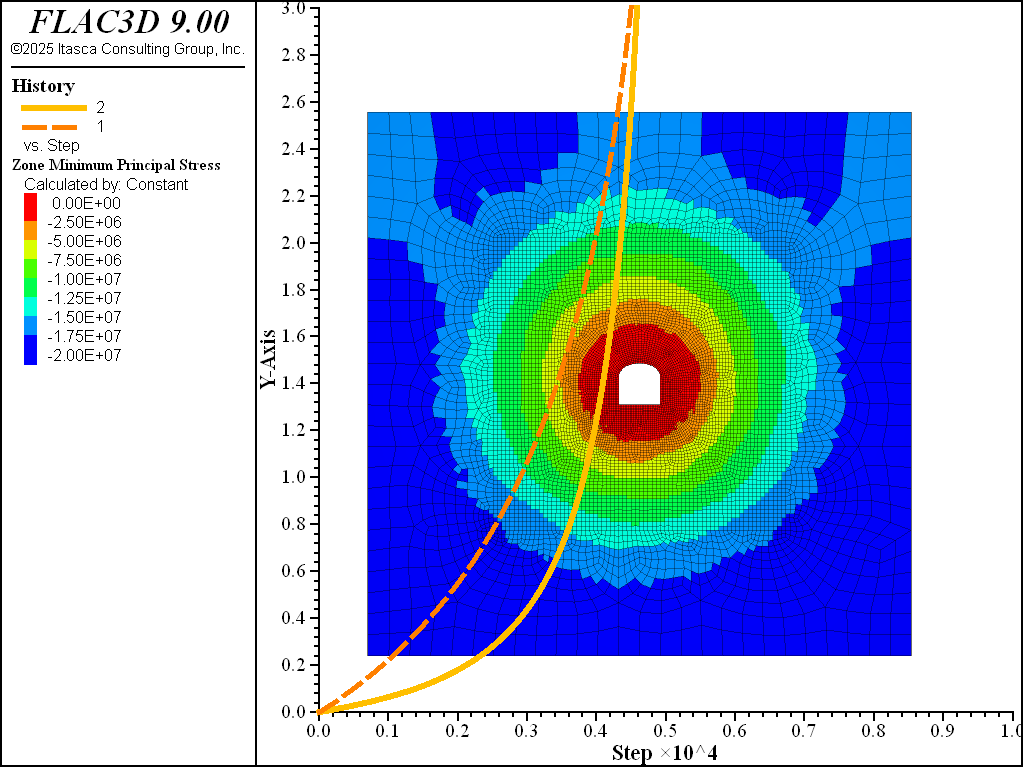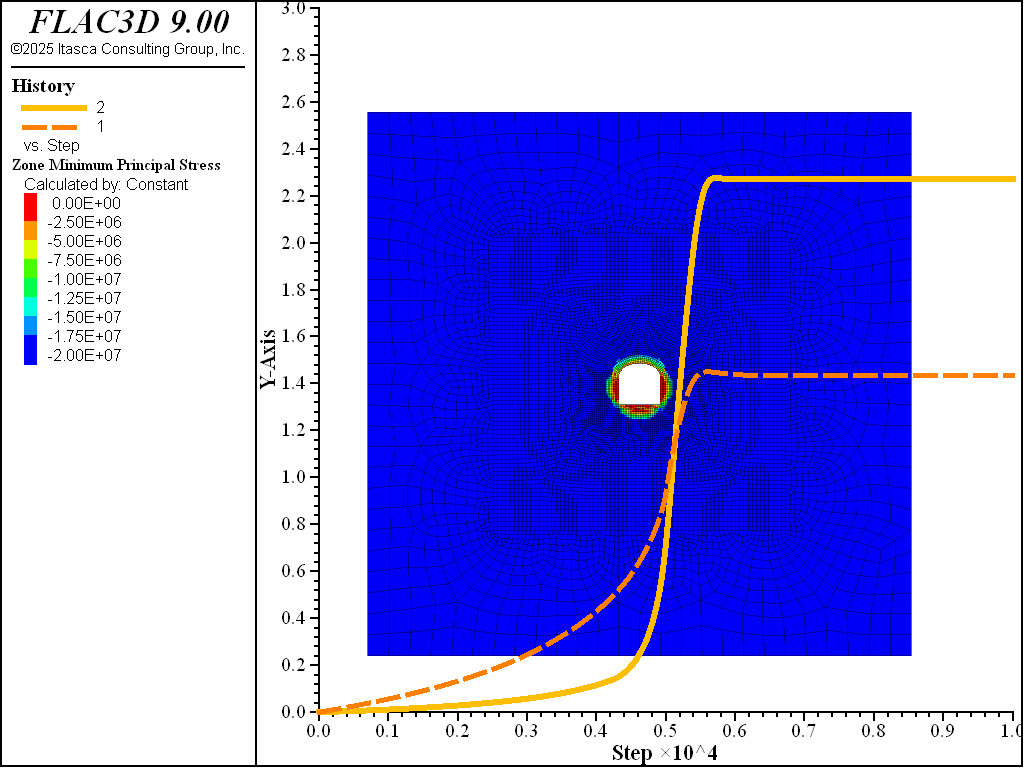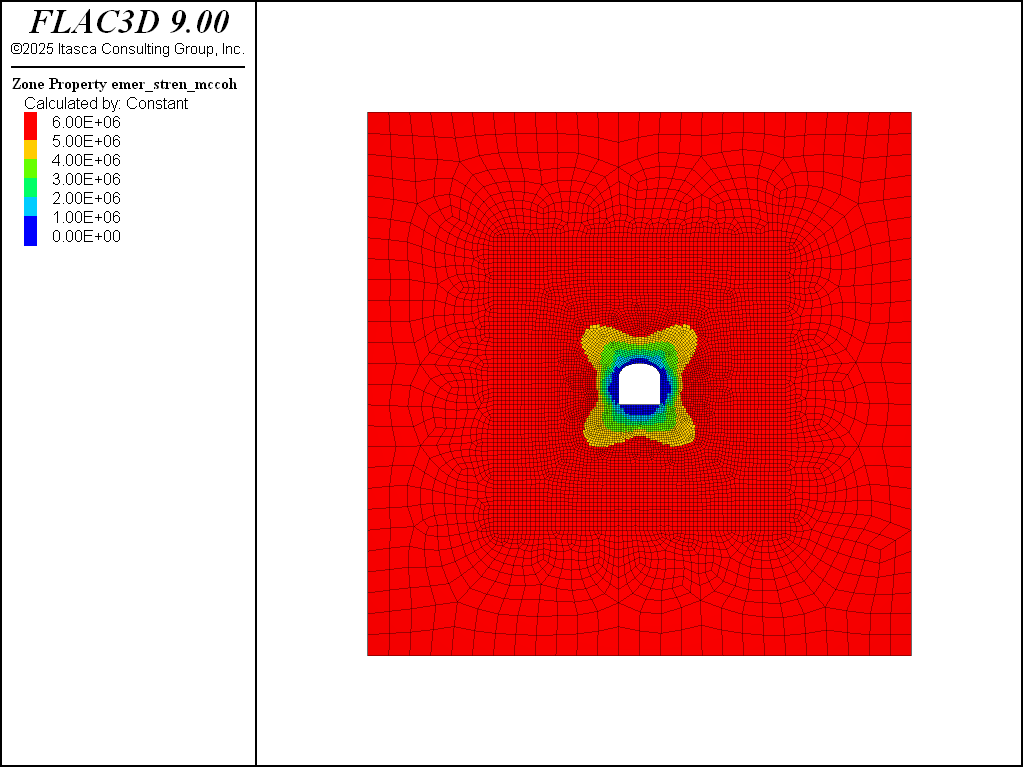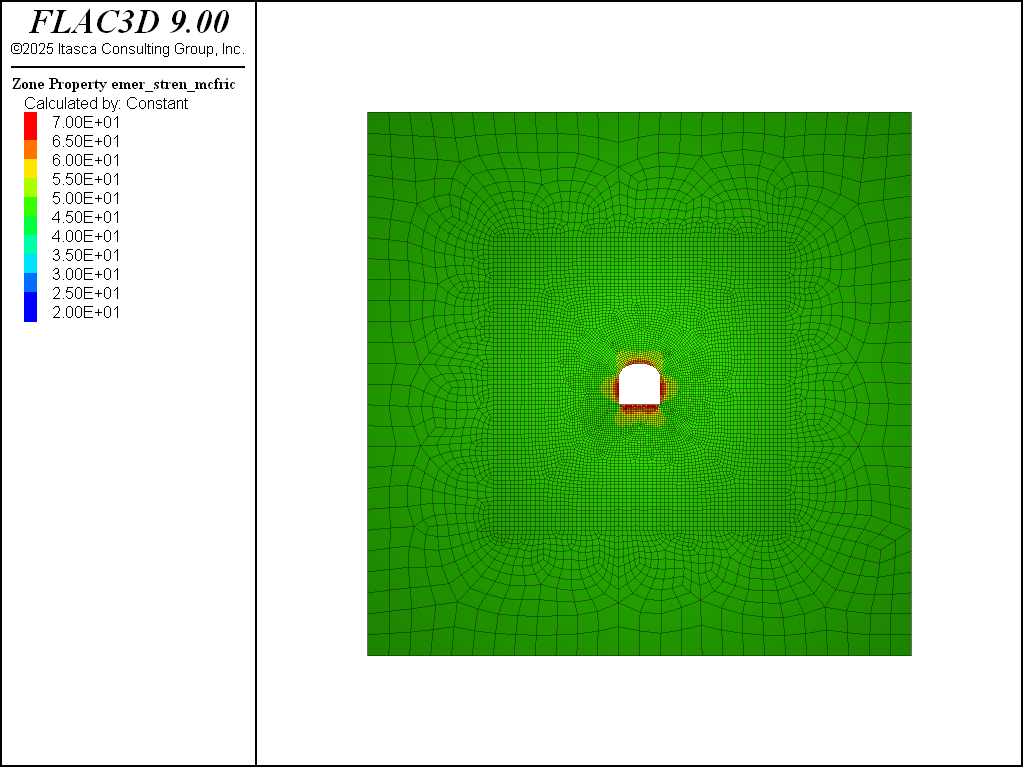FLAC3D Theory and Background • Constitutive Models

# Underground Tunnel Stability with IMASS Model

Note

To view this project in FLAC3D, use the menu command Help ► Examples…. Choose “Imass/ Tunnel” and select “tunnel.prj” to load. The project’s main data file is shown at the end of this example.

The stability of an underground opening is mainly driven by the ratio of in situ stress to the rock mass strength. Stability of a 5 m tunnel at 1000 m depth is simulated in this example for three rock mass qualities:

• Case 1: Very poor (GSI 30, UCS 20 MPa, mi 8 and Ei 17.4 GPa),

• Case 2: Moderate (GSI 45, UCS 60 MPa, mi 15 and Ei 25 GPa), and

• Case 3: Very good (GSI 75, UCS 150 MPa, mi 25 and Ei 51.4 GPa).

The results indicate that the tunnel in very poor quality rock mass in unstable while the tunnel in very good quality rock mass experiences minimal damage. The behavior of the tunnel excavated in moderate quality rock mass is somewhere in between. Now apart from rock mass strength, the purpose of this example is to highlight the importance of rock mass post-peak brittleness on the tunnel response in terms of depth of damage and convergence. Let’s consider the tunnel excavated in the moderate quality rock mass, and change the in_weak_multecrit (multiplier for critical strain) from 1.0 to 0.1 and 0.01. This will make the rock mass 10 and 100 times more brittle (transition from peak to post-peak envelope), respectively and basically the stresses will shed away faster from the yielded rock mass. Figure 1 shows the increase in depth of damage as well as tunnel closure with decreasing the critical strain. Figure 2 and Figure 3 show the associated loss of cohesion and mobilization of friction angle, respectively in the inner shell of the tunnel that is experiencing damage.Figure 1, Stress-strain response of the specimen for case 1, 2 and 3, respectively.Figure 2, Contour of instantaneous cohesion for case 1, 2 and 3, respectively.Figure 3, Contour of instantaneous friction angle for case 1, 2 and 3, respectively.

Data Files

tunnel1.dat

```model new
model large-strain off
model configure imass
model title 'Tunnel Stability Analysis'

; geometry
zone import 'grid.f3grid'
zone group 'Rock'
zone group 'Excavation' range group 'Block 1' slot 'Block'
zone face skin
; model properties
zone cmodel assign imass
zone property density 2500
zone property in_mod_youngintact 1.74e10
zone property in_stren_gsi 30.0
zone property in_stren_mi 8.0
zone property in_stren_ucsi 20e6
zone property in_weak_multecrit 1.0
; Boundary conditions
zone face apply velocity-y 0.0 range group 'North' or 'South'
zone face apply velocity-x 0.0 range group 'West' or 'East'
zone face apply velocity-z 0.0 range group 'Bottom' or 'Top'
; Initial stress
[global tunnelDepth = 1000.0]
[global zmax = 35.0]
[global grav = 9.81]
model gravity [grav]
zone initialize-stresses overburden [-(tunnelDepth-zmax)*grav*2500.0] ...
ratio 0.5 1.0 direction-x (1,0,0)
model solve elastic convergence 1
; Reset disp, vel and state
zone gridpoint initialize displacement (0,0,0)
zone gridpoint initialize velocity (0,0,0)
zone initialize state 0
;
fish define disp_average(list0,dof)
local dis0 = 0.0
local count0 = 0
loop foreach local gp0 list0
count0 = count0 + 1
dis0 += comp(gp.dis(gp0),dof)
endloop
dis0 /= float(count0)
return dis0
end
;
fish define disp_closure(list1, list2, dof)
local dis1 = disp_average(list1,dof)
local dis2 = disp_average(list2,dof)
return 100.0*(math.abs(dis1)+math.abs(dis2))/5.
end
;
[global gplist1 = zone.gp(zone.near(-0.0776873,0,1.92296))]
[global gplist2 = zone.gp(zone.near(5.08357,0,1.89728))]
[global gplist3 = zone.gp(zone.near(2.51578,0.25,-0.156949))]
[global gplist4 = zone.gp(zone.near(2.54146,0.25,5.10702))]
fish define v_closure
global h_closure = disp_closure(gplist1,gplist2,1)
global v_closure = disp_closure(gplist3,gplist4,3)
end
fish history v_closure
fish history h_closure
; Relaxation
zone relax excavate name 'Excavation' step 5000 ...
range group 'Excavation'
model solve cycle 10000
model save 'tunnel1'
```

tunnel2.dat

```model new
model large-strain off
model configure imass
model title 'Tunnel Stability Analysis'

; geometry
zone import 'grid.f3grid'
zone group 'Rock'
zone group 'Excavation' range group 'Block 1' slot 'Block'
zone face skin
; model properties
zone cmodel assign imass
zone property density 2500
zone property in_mod_youngintact 2.50e10
zone property in_stren_gsi 45.0
zone property in_stren_mi 15.0
zone property in_stren_ucsi 60e6
zone property in_weak_multecrit 1.0
; Boundary conditions
zone face apply velocity-y 0.0 range group 'North' or 'South'
zone face apply velocity-x 0.0 range group 'West' or 'East'
zone face apply velocity-z 0.0 range group 'Bottom' or 'Top'
; Initial stress
[global tunnelDepth = 1000.0]
[global zmax = 35.0]
[global grav = 9.81]
model gravity [grav]
zone initialize-stresses overburden [-(tunnelDepth-zmax)*grav*2500.0] ...
ratio 0.5 1.0 direction-x (1,0,0)
model solve elastic convergence 1
; Reset disp, vel and state
zone gridpoint initialize displacement (0,0,0)
zone gridpoint initialize velocity (0,0,0)
zone initialize state 0
;
fish define disp_average(list0,dof)
local dis0 = 0.0
local count0 = 0
loop foreach local gp0 list0
count0 = count0 + 1
dis0 += comp(gp.dis(gp0),dof)
endloop
dis0 /= float(count0)
return dis0
end
;
fish define disp_closure(list1, list2, dof)
local dis1 = disp_average(list1,dof)
local dis2 = disp_average(list2,dof)
return 100.0*(math.abs(dis1)+math.abs(dis2))/5.
end
;
[global gplist1 = zone.gp(zone.near(-0.0776873,0,1.92296))]
[global gplist2 = zone.gp(zone.near(5.08357,0,1.89728))]
[global gplist3 = zone.gp(zone.near(2.51578,0.25,-0.156949))]
[global gplist4 = zone.gp(zone.near(2.54146,0.25,5.10702))]
fish define v_closure
global h_closure = disp_closure(gplist1,gplist2,1)
global v_closure = disp_closure(gplist3,gplist4,3)
end
fish history v_closure
fish history h_closure
; Relaxation
zone relax excavate name 'Excavation' step 5000 ...
range group 'Excavation'
model solve cycle 10000
model save 'tunnel2'
```

tunnel3.dat

```model new
model large-strain off
model configure imass
model title 'Tunnel Stability Analysis'

; geometry
zone import 'grid.f3grid'
zone group 'Rock'
zone group 'Excavation' range group 'Block 1' slot 'Block'
zone face skin
; model properties
zone cmodel assign imass
zone property density 2500
zone property in_mod_youngintact 5.14e10
zone property in_stren_gsi 75.0
zone property in_stren_mi 25.0
zone property in_stren_ucsi 150e6
zone property in_weak_multecrit 1.0
; Boundary conditions
zone face apply velocity-y 0.0 range group 'North' or 'South'
zone face apply velocity-x 0.0 range group 'West' or 'East'
zone face apply velocity-z 0.0 range group 'Bottom' or 'Top'
; Initial stress
[global tunnelDepth = 1000.0]
[global zmax = 35.0]
[global grav = 9.81]
model gravity [grav]
zone initialize-stresses overburden [-(tunnelDepth-zmax)*grav*2500.0] ...
ratio 0.5 1.0 direction-x (1,0,0)
model solve elastic convergence 1
; Reset disp, vel and state
zone gridpoint initialize displacement (0,0,0)
zone gridpoint initialize velocity (0,0,0)
zone initialize state 0
;
fish define disp_average(list0,dof)
local dis0 = 0.0
local count0 = 0
loop foreach local gp0 list0
count0 = count0 + 1
dis0 += comp(gp.dis(gp0),dof)
endloop
dis0 /= float(count0)
return dis0
end
;
fish define disp_closure(list1, list2, dof)
local dis1 = disp_average(list1,dof)
local dis2 = disp_average(list2,dof)
return 100.0*(math.abs(dis1)+math.abs(dis2))/5.
end
;
[global gplist1 = zone.gp(zone.near(-0.0776873,0,1.92296))]
[global gplist2 = zone.gp(zone.near(5.08357,0,1.89728))]
[global gplist3 = zone.gp(zone.near(2.51578,0.25,-0.156949))]
[global gplist4 = zone.gp(zone.near(2.54146,0.25,5.10702))]
fish define v_closure
global h_closure = disp_closure(gplist1,gplist2,1)
global v_closure = disp_closure(gplist3,gplist4,3)
end
fish history v_closure
fish history h_closure
; Relaxation
zone relax excavate name 'Excavation' step 5000 ...
range group 'Excavation'
model solve cycle 10000
model save 'tunnel3'
```